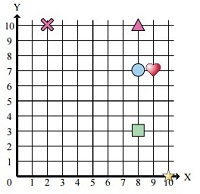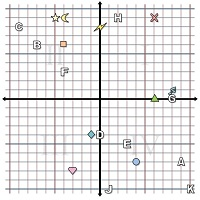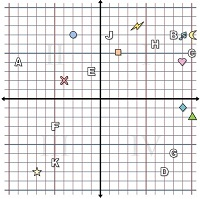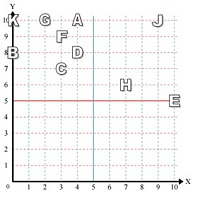# Reading a point in the coordinate plane Online Quiz

Following quiz provides Multiple Choice Questions (MCQs) related to Reading a point in the coordinate plane. You will have to read all the given answers and click over the correct answer. If you are not sure about the answer then you can check the answer using Show Answer button. You can use Next Quiz button to check new set of questions in the quiz.Q 1 - Use the grid below to determine the coordinates of the triangle shape.### Explanation

Reading from the grid, the x and y coordinates of the shape are 8 and 10 respectively. So the coordinates of the triangle shape are (8, 10)

Q 2 - Use the grid below to determine the coordinates of the letter E.### Explanation

Reading from the grid, the x and y coordinates of the letter are 3 and −5 respectively. So the coordinates of the letter E are (3, −5)

Q 3 - Use the grid below to determine the coordinates of the circle shape.### Explanation

Reading from the grid, the x and y coordinates of the shape are 4 and −7 respectively. So the coordinates of circle shape are (4, −7)

Q 4 - Use the grid below to determine the coordinates of the square shape.### Explanation

Reading from the grid, the x and y coordinates of the shape are 8 and 3 respectively. So the coordinates of square shape are (8, 3)

Q 5 - Use the grid below to determine the coordinates of the letter G.### Explanation

Reading from the grid, the x and y coordinates of the letter are 8 and −6 respectively. So the coordinates of letter G are (8, −6)

Q 6 - Use the grid below to determine the coordinates of the heart shape.### Explanation

Reading from the grid, the x and y coordinates of the shape are −3 and −8 respectively. So the coordinates of heart shape are (−3, −8)

Q 7 - Use the grid below to determine the coordinates of the letter B.### Explanation

Reading from the grid, the x and y coordinates of the letter are 8 and 7 respectively. So the coordinates of letter ‘B’ are (8, 7)

Q 8 - Use the grid below to determine the coordinates of the cross shape.### Explanation

Reading from the grid, the x and y coordinates of the cross shape are 2 and 10 respectively. So the coordinates of cross shape are (2, 10)

Q 9 - Use the grid below to determine the coordinates of the letter J.### Explanation

Reading from the grid, the x and y coordinates of the letter J are 1 and 7 respectively. So the coordinates of letter J are (1, 7)

Q 10 - Use the grid below to determine the coordinates of the letter C.